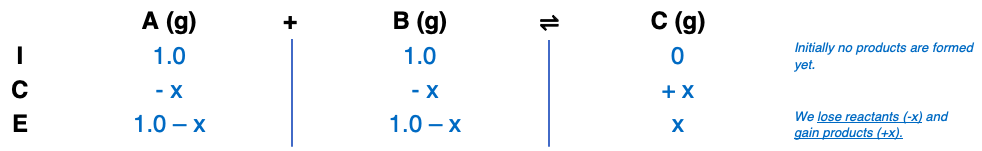# Problem: Consider the following reaction and associated equilibrium constant: aA(g) + bB(g) ⇌ cC(g); Kc = 4.0Find the equilibrium concentrations of A, B, and C for a = 1, b = 1, and c = 1. Assume that the initial concentrations of A and B are each 1.0 M and that no product is present at the beginning of the reaction.

🤓 Based on our data, we think this question is relevant for Professor Sharma's class at UM.

###### FREE Expert Solution###### Problem Details

Consider the following reaction and associated equilibrium constant: aA(g) + bB(g) ⇌ cC(g); Kc = 4.0

Find the equilibrium concentrations of A, B, and C for a = 1, b = 1, and c = 1. Assume that the initial concentrations of A and B are each 1.0 M and that no product is present at the beginning of the reaction.